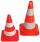# Right triangle + volume - math problems

#### Number of problems found: 190

• Tetrahedral pyramidA regular tetrahedral pyramid is given. Base edge length a = 6.5 cm, side edge s = 7.5 cm. Calculate the volume and the area of its face (side area).
• Rhombus baseCalculate the volume and surface area of prisms whose base is a rhombus with diagonals u1 = 12 cm and u2 = 15 cm. Prism height is twice the base edge length.
• Iglu - cone tentThe cone-shaped tent is 3 m high, the diameter of its base is 3.2 m. a) The tent is made of two layers of material. How many m2 of fabric is needed for production (including flooring) if 20% needs to be added to the minimum amount due to cutting waste? b)
• Digging a pitThe pit has the shape of a regular quadrilateral truncated pyramid. The edges of the bases are 14m and 10m long. The sidewalls form an angle of 135° with a smaller base. Determine how many m3 of soil were excavated when digging the pit?
• Vertical prismThe base of the vertical prism is a right triangle with leg a = 5 cm and a hypotenuse c = 13 cm. The height of the prism is equal to the circumference of the base. Calculate the surface area and volume of the prism
• Hexagonal pyramidCalculate the volume and the surface of a regular hexagonal pyramid with a base edge length of 3 cm and a height of 5 cm.
• Cylinder horizontallyThe cylinder with a diameter of 3 m and a height/length of 15 m is laid horizontally. Water is poured into it, reaching a height of 60 cm below the axis of the cylinder. How many hectoliters of water is in the cylinder?
• Tetrahedral pyramidCalculate the regular tetrahedral pyramid's volume and surface if the content area of the base is 20 cm2, and the deviation angle of the side edges from the plane of the base is 60 degrees.
• School modelThe beech school model of a regular quadrilateral pyramid has a base 20 cm long and 24 cm high. Calculate a) the surface of the pyramid in square decimeters, b) the mass of the pyramid in kilograms if the density of the beech is ρ = 0,8 g/cm ^ 3
• PrismCalculate the volume of the rhombic prism. The prism base is a rhombus whose one diagonal is 47 cm, and the edge of the base is 28 cm. The edge length of the base of the prism and height is 3:5.
• Rotary coneThe volume of the rotation of the cone is 472 cm3, and the angle between the side of the cone and the base angle is 70°. Calculate the lateral surface area of this cone.
• Children poolThe bottom of the children's pool is a regular hexagon with a = 60 cm side. The distance of opposing sides is 104 cm, the height of the pool is 45 cm. A) How many liters of water can fit into the pool? B) The pool is made of a double layer of plastic film
• Regular triangular pyramidCalculate the volume and surface area of the regular triangular pyramid and the height of the pyramid is 12 centimeters, the bottom edge has 4 centimeters and the height of the side wall is 12 centimeters
• Ratio-cuboidThe lengths of the edges of the cuboid are in the ratio 2: 3: 6. Its body diagonal is 14 cm long. Calculate the volume and surface area of the cuboid.
• Cone sideCalculate the volume and area of the cone whose height is 10 cm and the axial section of the cone has an angle of 30 degrees between height and the cone side.Calculate the volume (V) and the surface (S) of a regular quadrilateral prism whose height is 28.6 cm and the deviation of the body diagonal from the base plane is 50°.
• Traffic conesForty identical traffic cones with a base diameter d = 3 dm and a height v = 6 dm should be painted on the outside with orange paint (without base). How many crowns do we pay for color? If we need 50 cm ^ 3 of paint to paint, 1m2 and 1l of paint costs CZ
• Pine woodFrom a trunk of pine 6m long and 35 cm in diameter with a carved beam with a cross-section in the shape of a square so that the square had the greatest content area. Calculate the length of the sides of a square. Calculate the volume in cubic meters of lu
• Tetrahedral pyramid 8Let’s all side edges of the tetrahedral pyramid ABCDV be equally long and its base let’s be a rectangle. Determine its volume if you know the deviations A=40° B=70° of the planes of adjacent sidewalls and the plane of the base and the height h=16 of the p
• Four sided prismCalculate the volume and surface area of a regular quadrangular prism whose height is 28.6cm and the body diagonal forms a 50-degree angle with the base plane.

Do you have an exciting math question or word problem that you can't solve? Ask a question or post a math problem, and we can try to solve it.

We will send a solution to your e-mail address. Solved examples are also published here. Please enter the e-mail correctly and check whether you don't have a full mailbox.

Tip: Our volume units converter will help you with the conversion of volume units. See also our right triangle calculator. Right triangle Problems. Volume - math problems.